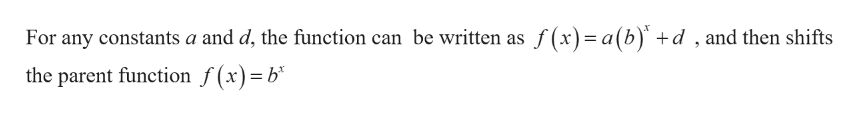# Give a formula for the function illustrated using a vertical shift and reflection of an exponential function. The two points marked on the graph are ?=(−1,51)A=(−1,51) and ?=(1,3)B=(1,3). The red horizontal line is given by ?=1y=1, and is a horizontal asymptote of the function.

Question
306 views

Give a formula for the function illustrated using a vertical shift and reflection of an exponential function. The two points marked on the graph are ?=(−1,51)A=(−1,51) and ?=(1,3)B=(1,3). The red horizontal line is given by ?=1y=1, and is a horizontal asymptote of the function.

check_circle

Step 1

Given,

Two points on the graph A=(−1,51) and B=(1,3) and a horizontal line y=1help_outlineImage TranscriptioncloseFor any constants a and d, the function can be written as f(x)= a(b) +d , and then shifts the parent function f(x)=b* fullscreen
Step 2

From the graph, the function has horizontal asymptote y=1. Therefore, the value of d is 1. Then

Step 3

Substitute the point A= (&minu...

### Want to see the full answer?

See Solution

#### Want to see this answer and more?

Solutions are written by subject experts who are available 24/7. Questions are typically answered within 1 hour.*

See Solution
*Response times may vary by subject and question.
Tagged in

### Other